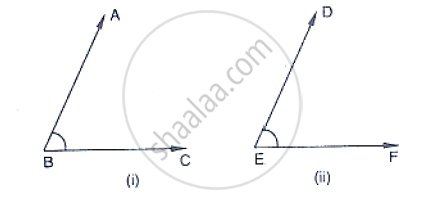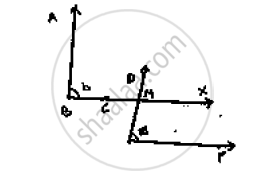# In the Below Fig, Arms Ba and Bc of ∠Abc Are Respectively Parallel to Arms Ed and Ef of ∠Def. Prove that ∠Abc = ∠Def. - Mathematics

In the below fig, arms BA and BC of ∠ABC are respectively parallel to arms ED and EF of
∠DEF. Prove that ∠ABC = ∠DEF.#### SolutionGiven AB || DE and BC ||lry  EF

To prove: ∠ABC = ∠DEF

Construction: Produce BC to x such that it intersects DE at M.

Proof: Since AB || DE and BX is the transversal

∴∠ABC = ∠DMX         [Corresponding angles]           ……..(ii)

Also,

BX || EF and DE is the transversal

∴∠DMX = ∠DEF     [Corresponding angles]           ……..(ii)

From (i) and (ii)

∴ABC = ∠DEF

Concept: Parallel Lines and a Transversal
Is there an error in this question or solution?

#### APPEARS IN

RD Sharma Mathematics for Class 9
Chapter 10 Lines and Angles
Exercise 10.4 | Q 26 | Page 49